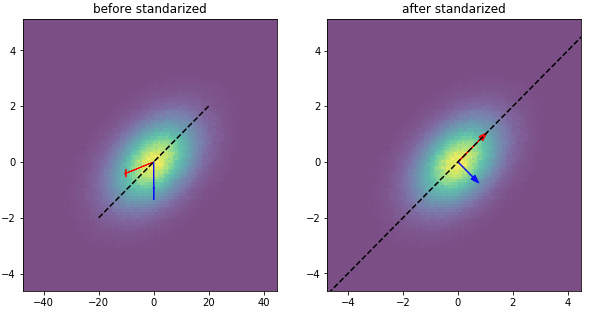# PCA降维之前为什么要先标准化？

统计/机器学习 数据预处理 数据降维    浏览次数：31873        分享

10机器小白   2017-03-21 13:25

##### 4个回答
31

PCA(主成分分析)所对应的数学理论是SVD(矩阵的奇异值分解）。而奇异值分解本身是完全不需要对矩阵中的元素做标准化或者去中心化的。

PCA通常是用于高维数据的降维，它可以将原来高维的数据投影到某个低维的空间上并使得其方差尽量大。如果数据其中某一特征（矩阵的某一列）的数值特别大，那么它在整个误差计算的比重上就很大，那么可以想象在投影到低维空间之后，为了使低秩分解逼近原数据，整个投影会去努力逼近最大的那一个特征，而忽略数值比较小的特征。因为在建模前我们并不知道每个特征的重要性，这很可能导致了大量的信息缺失。为了“公平”起见，防止过分捕捉某些数值大的特征，我们会对每个特征先进行标准化处理，使得它们的大小都在相同的范围内，然后再进行PCA。

SofaSofa数据科学社区DS面试题库 DS面经清风   2017-03-23 11:11

5#standarization of PCA
import numpy as np
import matplotlib.pyplot as plt
from sklearn.decomposition import PCA
from sklearn.preprocessing import StandardScaler
np.set_printoptions(precision=3)
np.random.seed(0)
n=1000000
mu=[0,0]
corr=np.array([[1.,.4],[.4,1.]])
std_vector=[10.,1]

A_ori=np.random.multivariate_normal(mu,corr,n)
A_scaled=np.matmul(A_ori,np.diag(std_vector))
scaler = StandardScaler()
scaler.fit(A_scaled)
A_standarized=scaler.transform(A_scaled)

pca = PCA()
pca.fit(A_scaled)
pca1 = PCA()
pca1.fit(A_standarized)

print('Correlation Coefficient matrix is:')
print(corr)
print('std vector is:')
print(std_vector)

print('Covariance matrix is:')
print(np.cov(A_scaled.T))

print('---Before standarization---')
print('Components:')
print(pca.components_)
print('Sigular values:')
print(pca.explained_variance_)

print('---After standarization---')
print('Components:')
print(pca1.components_)
print('Sigular values:')
print(pca1.explained_variance_)

# draw PCA components
t1=np.linspace(-20,20,100)
t2=t1*std_vector/std_vector

plt.figure(figsize=[10,5])
plt.subplot(121)
plt.hist2d(A_scaled[:,0],A_scaled[:,1],100,alpha=0.7)
plt.plot(t1,t2,'--k')
c=pca.components_
r=np.sqrt(pca.explained_variance_)
# plt.axis('equal')
plt.title('before standarized')

t1=np.linspace(-20,20,100)
cov=np.cov(A_standarized.T)
t2=t1*cov[1,1]
plt.subplot(122)
plt.hist2d(A_standarized[:,0],A_standarized[:,1],100,alpha=0.7)
plt.plot(t1,t2,'--k')
c=pca1.components_
r=np.sqrt(pca1.explained_variance_)
# plt.axis('equal')
plt.title('after standarized')
plt.show()
SofaSofa数据科学社区DS面试题库 DS面经Zealing   2019-04-30 21:17

2

PCA实现的方式其实有四种：

• 标准化数据后的协方差矩阵
• 标准化数据后的相关系数矩阵
• 未标准化数据后的相关系数矩阵
• 标准化数据后的svd方式

SofaSofa数据科学社区DS面试题库 DS面经zhanglu   2018-03-28 12:58

1

SofaSofa数据科学社区DS面试题库 DS面经奶瓶他哥   2019-04-26 16:33相关讨论随便看看